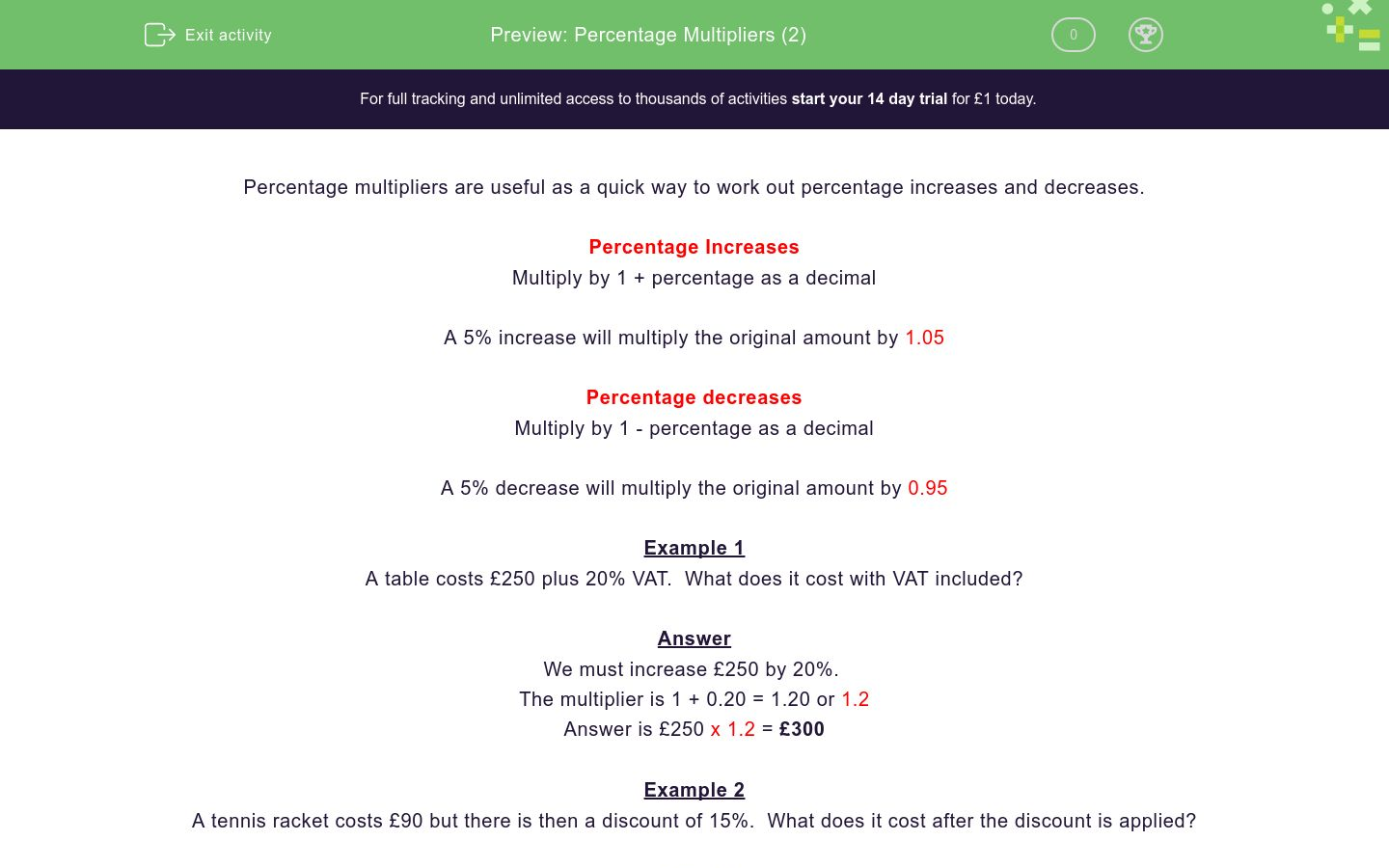# Percentage Multipliers (2)

In this worksheet, students use percentage multipliers to work out the final price after a percentage increase or decrease.Key stage:  KS 4

Curriculum topic:  Ratio, Proportion and Rates of Change

Curriculum subtopic:  Set Up, Solve and Interpret Answers in Growth and Decay Problems

Difficulty level:### QUESTION 1 of 10

Percentage multipliers are useful as a quick way to work out percentage increases and decreases.

Percentage Increases

Multiply by 1 + percentage as a decimal

A 5% increase will multiply the original amount by 1.05

Percentage decreases

Multiply by 1 - percentage as a decimal

A 5% decrease will multiply the original amount by 0.95

Example 1

A table costs £250 plus 20% VAT.  What does it cost with VAT included?

Answer

We must increase £250 by 20%.

The multiplier is 1 + 0.20 = 1.20 or 1.2

Answer is £250 x 1.2 = £300

Example 2

A tennis racket costs £90 but there is then a discount of 15%.  What does it cost after the discount is applied?

Answer

We must decrease £90 by 15%.

The multiplier is 1 - 0.15 = 0.85

Answer is £90 x 0.85 = £76.50

Use a percentage multiplier to calculate the final price.

A child's car seat costs £65 + 5% VAT.

£65.50

£61.75

£68.25

£97.50

Use a percentage multiplier to calculate the final price.

A car costs £9500 + 20% VAT.

£9520

£1900

£11250

£11400

Use a percentage multiplier to calculate the final price.

A football costs £12 less a 10% discount

£11.90

£10

£10.80

£11

Use a percentage multiplier to calculate the final price.

A suitcase costs £48 less a 25% discount

£12

£36

£60

£47.75

Use a percentage multiplier to calculate the final price.

A bicycle costs £150 + 20% VAT.

£120

£180

£150.20

£152

Use a percentage multiplier to calculate the final price.

A leather handbag costs €80 less a 12% discount

€70.40

€70.04

€68

€89.60

Use a percentage multiplier to calculate the final price.

A motorbike costs €2500 + 22% VAT.

€3050

€2522

€1950

€3000

Use a percentage multiplier to calculate the final price.

A clock costs €145 + 15% VAT.

€175

€168.25

€166.75

€170

Use a percentage multiplier to calculate the final price.

A book costs £15 less an 8% discount

£12

£12.50

£13.08

£13.80

Use a percentage multiplier to calculate the final price.

A picture costs £74 less a 12½% discount

£83.25

£64.75

£63

£65.25

• Question 1

Use a percentage multiplier to calculate the final price.

A child's car seat costs £65 + 5% VAT.

CORRECT ANSWER
£68.25
EDDIE SAYS
£65 × 1.05
• Question 2

Use a percentage multiplier to calculate the final price.

A car costs £9500 + 20% VAT.

CORRECT ANSWER
£11400
EDDIE SAYS
£9500 × 1.2
• Question 3

Use a percentage multiplier to calculate the final price.

A football costs £12 less a 10% discount

CORRECT ANSWER
£10.80
EDDIE SAYS
£12 × 0.9
• Question 4

Use a percentage multiplier to calculate the final price.

A suitcase costs £48 less a 25% discount

CORRECT ANSWER
£36
EDDIE SAYS
£48 × 0.75
• Question 5

Use a percentage multiplier to calculate the final price.

A bicycle costs £150 + 20% VAT.

CORRECT ANSWER
£180
EDDIE SAYS
£150 × 1.2
• Question 6

Use a percentage multiplier to calculate the final price.

A leather handbag costs €80 less a 12% discount

CORRECT ANSWER
€70.40
EDDIE SAYS
€80 × 0.88
• Question 7

Use a percentage multiplier to calculate the final price.

A motorbike costs €2500 + 22% VAT.

CORRECT ANSWER
€3050
EDDIE SAYS
€2500 × 1.22
• Question 8

Use a percentage multiplier to calculate the final price.

A clock costs €145 + 15% VAT.

CORRECT ANSWER
€166.75
EDDIE SAYS
€145 × 1.15
• Question 9

Use a percentage multiplier to calculate the final price.

A book costs £15 less an 8% discount

CORRECT ANSWER
£13.80
EDDIE SAYS
£15 × 0.92
• Question 10

Use a percentage multiplier to calculate the final price.

A picture costs £74 less a 12½% discount

CORRECT ANSWER
£64.75
EDDIE SAYS
£74 × 0.875
---- OR ----

Sign up for a £1 trial so you can track and measure your child's progress on this activity.

### What is EdPlace?

We're your National Curriculum aligned online education content provider helping each child succeed in English, maths and science from year 1 to GCSE. With an EdPlace account you’ll be able to track and measure progress, helping each child achieve their best. We build confidence and attainment by personalising each child’s learning at a level that suits them.

Start your £1 trial